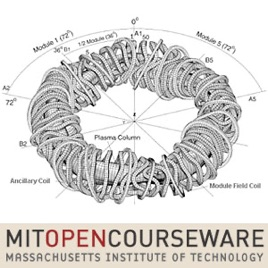12 Folgen

This video course covers the fundamental concepts and computer implementations of finite element analysis for linear systems, with examples taken from nuclear, civil, mechanical, aeronautical, and other fields of engineering.# Finite Element Procedures for Solids and Structures, Linear Analysis MIT

• Technologie

This video course covers the fundamental concepts and computer implementations of finite element analysis for linear systems, with examples taken from nuclear, civil, mechanical, aeronautical, and other fields of engineering.

• video
Lecture 1: Some basic concepts of engineering analysis

## Lecture 1: Some basic concepts of engineering analysis

This introductory video discusses the use of finite element models in engineering, focusing on translating physical situations into systems of equations. Problems are classified as discrete or continuous, and static, dynamic, or eigenvalue.

• 45 Min.
• video
Lecture 2: Analysis of continuous systems

## Lecture 2: Analysis of continuous systems

This lecture introduces the differential and variational formulations for continuous systems. Examples show how to use boundary conditions, the principle of virtual displacements, weighted residual methods (Galerkin, least squares) and the Ritz method.

• 58 Min.
• video
Lecture 3: The displacement-based finite element method

## Lecture 3: The displacement-based finite element method

The displacement-based finite element method uses matrices to represent the external forces, stiffnesses, and resulting displacement of each 3D finite element. Interpolation matrices and boundary conditions for dynamic analysis are also discussed.

• 57 Min.
• video
Lecture 4: Generalized coordinate finite element models

## Lecture 4: Generalized coordinate finite element models

Specific problem types and their displacement, strain, and stress matrices are described: truss, beam, plate, shell, plane stress or strain, and axisymmetric systems. Other topics include convergence of solutions and several categories of error analysis.

• 56 Min.
• video
Lecture 5: Implementation of Methods in Computer Programs

## Lecture 5: Implementation of Methods in Computer Programs

Details of how to implement the finite element method in software are given, using the example of a cantilever plate. Practical 1D, 2D, and 3D variable-number-node elements are introduced for the common systems described in lecture 4.

• 55 Min.
• video
Lecture 6: Formulation and calculation of isoparametric models

## Lecture 6: Formulation and calculation of isoparametric models

Isoparametric elements provide an alternative to the generalized coordinate elements discussed in lecture 4, interpolating both the element displacements and geometry. This lecture focuses on continuum elements.

• 56 Min.